##### The Japanese Bonsai specialist
Direct order Contact Help / Services Newsletter# Granite stone lantern nishinoya 150 cm

Nishinoya toro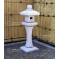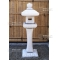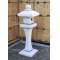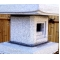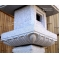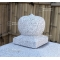ref. : 9376

830,00

voluminous/heavy item extra shipping of 55,00

Available quantity : 1Order

###### Description

Height 150 cm, top section 45*45 cm, weight 330 kilograms, delivered in 6 elements. This solid granite lantern is a rare model in Europe since it’s a copy of a toro from a garden in Kyoto. The reflecting lotus at the base is joined by a sinuous pole that leads up to a supporting lotus and compartment with framed square windows. A bulb can be added to illuminate it, lighting pathways and entrances.

#lantern 5.7 #stone 4.5 #granite 3.7 #nishinoya 2.9 #lotus 2.5 #compartment 2.1 #supporting 2 #reflecting 2 #illuminate 2 #kilograms 1.9

Formule
(( ROUND((CHAR_LENGTH(b.article_nom)-CHAR_LENGTH(REPLACE(b.article_nom, 'granite', '')))/LENGTH('granite')) + ROUND((CHAR_LENGTH(b.article_description)-CHAR_LENGTH(REPLACE(b.article_description, 'granite', '')))/LENGTH('granite')) ) * 3.7) + (( ROUND((CHAR_LENGTH(b.article_nom)-CHAR_LENGTH(REPLACE(b.article_nom, 'lantern', '')))/LENGTH('lantern')) + ROUND((CHAR_LENGTH(b.article_description)-CHAR_LENGTH(REPLACE(b.article_description, 'lantern', '')))/LENGTH('lantern')) ) * 3.7) + (( ROUND((CHAR_LENGTH(b.article_nom)-CHAR_LENGTH(REPLACE(b.article_nom, 'nishinoya', '')))/LENGTH('nishinoya')) + ROUND((CHAR_LENGTH(b.article_description)-CHAR_LENGTH(REPLACE(b.article_description, 'nishinoya', '')))/LENGTH('nishinoya')) ) * 2.9) + (( ROUND((CHAR_LENGTH(b.article_nom)-CHAR_LENGTH(REPLACE(b.article_nom, 'lotus', '')))/LENGTH('lotus')) + ROUND((CHAR_LENGTH(b.article_description)-CHAR_LENGTH(REPLACE(b.article_description, 'lotus', '')))/LENGTH('lotus')) ) * 2.5) + (( ROUND((CHAR_LENGTH(b.article_nom)-CHAR_LENGTH(REPLACE(b.article_nom, 'stone', '')))/LENGTH('stone')) + ROUND((CHAR_LENGTH(b.article_description)-CHAR_LENGTH(REPLACE(b.article_description, 'stone', '')))/LENGTH('stone')) ) * 2.5) + (( ROUND((CHAR_LENGTH(b.article_nom)-CHAR_LENGTH(REPLACE(b.article_nom, 'compartment', '')))/LENGTH('compartment')) + ROUND((CHAR_LENGTH(b.article_description)-CHAR_LENGTH(REPLACE(b.article_description, 'compartment', '')))/LENGTH('compartment')) ) * 2.1) + (( ROUND((CHAR_LENGTH(b.article_nom)-CHAR_LENGTH(REPLACE(b.article_nom, 'supporting', '')))/LENGTH('supporting')) + ROUND((CHAR_LENGTH(b.article_description)-CHAR_LENGTH(REPLACE(b.article_description, 'supporting', '')))/LENGTH('supporting')) ) * 2) + (( ROUND((CHAR_LENGTH(b.article_nom)-CHAR_LENGTH(REPLACE(b.article_nom, 'reflecting', '')))/LENGTH('reflecting')) + ROUND((CHAR_LENGTH(b.article_description)-CHAR_LENGTH(REPLACE(b.article_description, 'reflecting', '')))/LENGTH('reflecting')) ) * 2) + (( ROUND((CHAR_LENGTH(b.article_nom)-CHAR_LENGTH(REPLACE(b.article_nom, 'illuminate', '')))/LENGTH('illuminate')) + ROUND((CHAR_LENGTH(b.article_description)-CHAR_LENGTH(REPLACE(b.article_description, 'illuminate', '')))/LENGTH('illuminate')) ) * 2) + (( ROUND((CHAR_LENGTH(b.article_nom)-CHAR_LENGTH(REPLACE(b.article_nom, 'kilograms', '')))/LENGTH('kilograms')) + ROUND((CHAR_LENGTH(b.article_description)-CHAR_LENGTH(REPLACE(b.article_description, 'kilograms', '')))/LENGTH('kilograms')) ) * 1.9)

## Secure payment## Delivery

Our logistic partners :